# Dipole

﻿
Dipole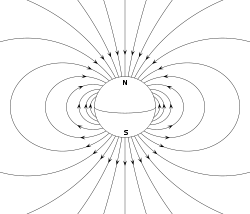The Earth's magnetic field, approximated as a magnetic dipole. However, the "N" and "S" (north and south) poles are labeled here geographically, which is the opposite of the convention for labeling the poles of a magnetic dipole moment

In physics, there are several kinds of dipoles:

• An electric dipole is a separation of positive and negative charges. The simplest example of this is a pair of electric charges of equal magnitude but opposite sign, separated by some (usually small) distance. A permanent electric dipole is called an electret.
• A magnetic dipole is a closed circulation of electric current. A simple example of this is a single loop of wire with some constant current flowing through it.
• A flow dipole is a separation of a sink and a source. In a highly viscous medium, a two-beater kitchen mixer causes a dipole flow field.
• An acoustic dipole is the oscillating version of it. A simple example is a dipole speaker.
• Any scalar or other field may have a dipole moment.

Dipoles can be characterized by their dipole moment, a vector quantity. For the simple electric dipole given above, the electric dipole moment points from the negative charge towards the positive charge, and has a magnitude equal to the strength of each charge times the separation between the charges. (To be precise: for the definition of the dipole moment one should always consider the "dipole limit", where e.g. the distance of the generating charges should converge to 0, while simultaneously the charge strength should diverge to infinity in such a way that the product remains a positive constant.)

For the current loop, the magnetic dipole moment points through the loop (according to the right hand grip rule), with a magnitude equal to the current in the loop times the area of the loop.

In addition to current loops, the electron, among other fundamental particles, has a magnetic dipole moment. This is because it generates a magnetic field that is identical to that generated by a very small current loop. However, to the best of our knowledge, the electron's magnetic moment is not due to a current loop, but is instead an intrinsic property of the electron. It is also possible that the electron has an electric dipole moment, although this has not yet been observed (see electron electric dipole moment for more information).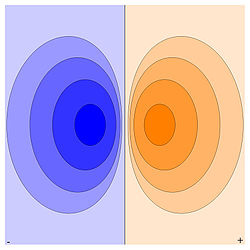Contour plot of the electrostatic potential of a horizontally oriented electrical dipole of finite size. Strong colors indicate highest and lowest potential (where the opposing charges of the dipole are located).

A permanent magnet, such as a bar magnet, owes its magnetism to the intrinsic magnetic dipole moment of the electron. The two ends of a bar magnet are referred to as poles (not to be confused with monopoles), and are labeled "north" and "south." The dipole moment of the bar magnet points from its magnetic south to its magnetic north pole. The north pole of a bar magnet in a compass points north. However, this means that Earth's geomagnetic north pole is the south pole of its dipole moment, and vice versa.

The only known mechanisms for the creation of magnetic dipoles are by current loops or quantum-mechanical spin since the existence of magnetic monopoles has never been experimentally demonstrated.

The term comes from the Greek δίς (dis), "twice" and πόλος (pòlos), "axis".

## Classification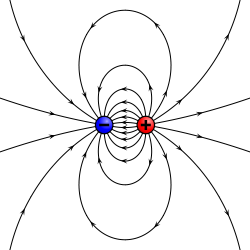Electric field lines of two opposing charges separated by a finite distance.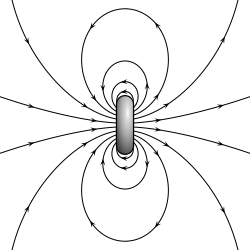Magnetic field lines of a ring current of finite diameter.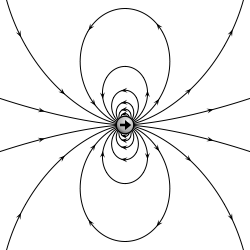Field lines of a point dipole of any type, electric, magnetic, acoustic, …

A physical dipole consists of two equal and opposite point charges: in the literal sense, two poles. Its field at large distances (i.e., distances large in comparison to the separation of the poles) depends almost entirely on the dipole moment as defined above. A point (electric) dipole is the limit obtained by letting the separation tend to 0 while keeping the dipole moment fixed. The field of a point dipole has a particularly simple form, and the order-1 term in the multipole expansion is precisely the point dipole field.

Although there are no known magnetic monopoles in nature, there are magnetic dipoles in the form of the quantum-mechanical spin associated with particles such as electrons (although the accurate description of such effects falls outside of classical electromagnetism). A theoretical magnetic point dipole has a magnetic field of exactly the same form as the electric field of an electric point dipole. A very small current-carrying loop is approximately a magnetic point dipole; the magnetic dipole moment of such a loop is the product of the current flowing in the loop and the (vector) area of the loop.

Any configuration of charges or currents has a 'dipole moment', which describes the dipole whose field is the best approximation, at large distances, to that of the given configuration. This is simply one term in the multipole expansion when the total charge ("monopole moment") is 0 — as it always is for the magnetic case, since there are no magnetic monopoles. The dipole term is the dominant one at large distances: Its field falls off in proportion to 1/r3, as compared to 1/r4 for the next (quadrupole) term and higher powers of 1/r for higher terms, or 1/r2 for the monopole term.

## Molecular dipoles

Many molecules have such dipole moments due to non-uniform distributions of positive and negative charges on the various atoms. Such is the case with polar compounds like hydrogen fluoride (HF), where electron density is shared unequally between atoms.

A molecule with a permanent dipole moment is called a polar molecule. A molecule is polarized when it carries an induced dipole. The physical chemist Peter J. W. Debye was the first scientist to study molecular dipoles extensively, and, as a consequence, dipole moments are measured in units named debye in his honor.

With respect to molecules, there are three types of dipoles:

• Permanent dipoles: These occur when two atoms in a molecule have substantially different electronegativity: One atom attracts electrons more than another, becoming more negative, while the other atom becomes more positive. See dipole-dipole attractions.
• Instantaneous dipoles: These occur due to chance when electrons happen to be more concentrated in one place than another in a molecule, creating a temporary dipole. See instantaneous dipole.
• Induced dipoles: These can occur when one molecule with a permanent dipole repels another molecule's electrons, "inducing" a dipole moment in that molecule. See induced-dipole attraction.

More generally, an induced dipole of any polarizable charge distribution ρ (remember that a molecule has a charge distribution) is caused by an electric field external to ρ. This field may, for instance, originate from an ion or polar molecule in the vicinity of ρ or may be macroscopic (e.g., a molecule between the plates of a charged capacitor). The size of the induced dipole is equal to the product of the strength of the external field and the dipole polarizability of ρ.

Typical gas phase values of some chemical compounds in debye units:

These values can be obtained from measurement of the dielectric constant. When the symmetry of a molecule cancels out a net dipole moment, the value is set at 0. The highest dipole moments are in the range of 10 to 11. From the dipole moment information can be deduced about the molecular geometry of the molecule. For example the data illustrate that carbon dioxide is a linear molecule but ozone is not.

## Quantum mechanical dipole operator

Consider a collection of N particles with charges qi and position vectors ri. For instance, this collection may be a molecule consisting of electrons, all with charge −e, and nuclei with charge eZi, where Zi is the atomic number of the i th nucleus. The physical quantity (observable) dipole has the quantum mechanical dipole operator:$\mathfrak{p} = \sum_{i=1}^N \, q_i \, \mathbf{r}_i \, .$

## Atomic dipoles

A non-degenerate (S-state) atom can have only a zero permanent dipole. This fact follows quantum mechanically from the inversion symmetry of atoms. All 3 components of the dipole operator are antisymmetric under inversion with respect to the nucleus,$\mathfrak{I} \;\mathfrak{p}\; \mathfrak{I}^{-1} = - \mathfrak{p},$

where$\stackrel{\mathfrak{p}}{}$ is the dipole operator and$\stackrel{\mathfrak{I}}{}\,$ is the inversion operator. The permanent dipole moment of an atom in a non-degenerate state (see degenerate energy level) is given as the expectation (average) value of the dipole operator,$\langle \mathfrak{p} \rangle = \langle\, S\, | \mathfrak{p} |\, S \,\rangle,$

where$|\, S\, \rangle$ is an S-state, non-degenerate, wavefunction, which is symmetric or antisymmetric under inversion:$\mathfrak{I}\,|\, S\, \rangle= \pm |\, S\, \rangle$. Since the product of the wavefunction (in the ket) and its complex conjugate (in the bra) is always symmetric under inversion and its inverse,$\langle \mathfrak{p} \rangle = \langle\, \mathfrak{I}^{-1}\, S\, | \mathfrak{p} |\, \mathfrak{I}^{-1}\, S \,\rangle = \langle\, S\, | \mathfrak{I}\, \mathfrak{p} \, \mathfrak{I}^{-1}| \, S \,\rangle = -\langle \mathfrak{p} \rangle$

it follows that the expectation value changes sign under inversion. We used here the fact that$\mathfrak{I}\,$, being a symmetry operator, is unitary:$\mathfrak{I}^{-1} = \mathfrak{I}^{*}\,$ and by definition the Hermitian adjoint$\mathfrak{I}^*\,$ may be moved from bra to ket and then becomes$\mathfrak{I}^{**} = \mathfrak{I}\,$. Since the only quantity that is equal to minus itself is the zero, the expectation value vanishes,$\langle \mathfrak{p}\rangle = 0.$

In the case of open-shell atoms with degenerate energy levels, one could define a dipole moment by the aid of the first-order Stark effect. This gives a non-vanishing dipole (by definition proportional to a non-vanishing first-order Stark shift) only if some of the wavefunctions belonging to the degenerate energies have opposite parity; i.e., have different behavior under inversion. This is a rare occurrence, but happens for the excited H-atom, where 2s and 2p states are "accidentally" degenerate (see this article for the origin of this degeneracy) and have opposite parity (2s is even and 2p is odd).

## Field of a static magnetic dipole

### Magnitude

The far-field strength, B, of a dipole magnetic field is given by$B(m, r, \lambda) = \frac {\mu_0} {4\pi} \frac {m} {r^3} \sqrt {1+3\sin^2\lambda} \, ,$

where

B is the strength of the field, measured in teslas
r is the distance from the center, measured in metres
λ is the magnetic latitude (equal to 90° − θ) where θ is the magnetic colatitude, measured in radians or degrees from the dipole axis[note 1]
m is the dipole moment (VADM=virtual axial dipole moment), measured in ampere square-metres (A·m2), which equals joules per tesla
μ0 is the permeability of free space, measured in henries per metre.

Conversion to cylindrical coordinates is achieved using r2 = z2 + ρ2 and$\lambda = \arcsin\left(\frac{z}{\sqrt{z^2+\rho^2}}\right)$

where ρ is the perpendicular distance from the z-axis. Then,$B(\rho,z) = \frac{\mu_0 m}{4 \pi (z^2+\rho^2)^{3/2}} \sqrt{1+\frac{3 z^2}{z^2 + \rho^2}}$

### Vector form

The field itself is a vector quantity:$\mathbf{B}(\mathbf{m}, \mathbf{r}) = \frac {\mu_0} {4\pi r^3} \left(3(\mathbf{m}\cdot\hat{\mathbf{r}})\hat{\mathbf{r}}-\mathbf{m}\right) + \frac{2\mu_0}{3}\mathbf{m}\delta^3(\mathbf{r})$

where

B is the field
r is the vector from the position of the dipole to the position where the field is being measured
r is the absolute value of r: the distance from the dipole$\hat{\mathbf{r}} = \mathbf{r}/r$ is the unit vector parallel to r;
m is the (vector) dipole moment
μ0 is the permeability of free space
δ3 is the three-dimensional delta function.[note 2]

This is exactly the field of a point dipole, exactly the dipole term in the multipole expansion of an arbitrary field, and approximately the field of any dipole-like configuration at large distances.

### Magnetic vector potential

The vector potential A of a magnetic dipole is$\mathbf{A}(\mathbf{r}) = \frac {\mu_0} {4\pi r^2} (\mathbf{m}\times\hat{\mathbf{r}})$

with the same definitions as above.

## Field from an electric dipole

The electrostatic potential at position r due to an electric dipole at the origin is given by:$\Phi(\mathbf{r}) = \frac{1}{4\pi\varepsilon_0}\,\frac{\mathbf{p}\cdot\hat{\mathbf{r}}}{r^2}$

where$\hat{\mathbf{r}}$ is a unit vector in the direction of r', p is the (vector) dipole moment, and ε0 is the permittivity of free space.

This term appears as the second term in the multipole expansion of an arbitrary electrostatic potential Φ(r). If the source of Φ(r) is a dipole, as it is assumed here, this term is the only non-vanishing term in the multipole expansion of Φ(r). The electric field from a dipole can be found from the gradient of this potential:$\mathbf{E} = - \nabla \Phi =\frac {1} {4\pi\epsilon_0} \left(\frac{3(\mathbf{p}\cdot\hat{\mathbf{r}})\hat{\mathbf{r}}-\mathbf{p}}{r^3}\right) - \frac{1}{3\epsilon_0}\mathbf{p}\delta^3(\mathbf{r})$

where E is the electric field and δ3 is the 3-dimensional delta function.[note 2] This is formally identical to the magnetic field of a point magnetic dipole; only a few names have changed.

## Torque on a dipole

Since the direction of an electric field is defined as the direction of the force on a positive charge, electric field lines point away from a positive charge and toward a negative charge.

When placed in an electric or magnetic field, equal but opposite forces arise on each side of the dipole creating a torque τ:$\boldsymbol{\tau} = \mathbf{p} \times \mathbf{E}$

for an electric dipole moment p (in coulomb-meters), or$\boldsymbol{\tau} = \mathbf{m} \times \mathbf{B}$

for a magnetic dipole moment m (in ampere-square meters).

The resulting torque will tend to align the dipole with the applied field, which in the case of an electric dipole, yields a potential energy of$U = -\mathbf{p} \cdot \mathbf{E}$.

The energy of a magnetic dipole is similarly$U = -\mathbf{m} \cdot \mathbf{B}$.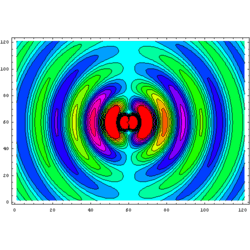Real-time evolution of the magnetic field of an oscillating electric dipole. The field lines, which are horizontal rings around the axis of the vertically oriented dipole, are perpendicularly crossing the x-y-plane of the image. Shown as a colored contour plot is the z-component of the field. Cyan is zero magnitude, green–yellow–red and blue–pink–red are increasing strengths in opposing directions.

In addition to dipoles in electrostatics, it is also common to consider an electric or magnetic dipole that is oscillating in time.

In particular, a harmonically oscillating electric dipole is described by a dipole moment of the form$\mathbf{p}=\mathbf{p'(\mathbf r)}e^{-i\omega t} \, ,$

where ω is the angular frequency. In vacuum, this produces fields:$\mathbf{E} = \frac{1}{4\pi\varepsilon_0} \left\{ \frac{\omega^2}{c^2 r} ( \hat{\mathbf{r}} \times \mathbf{p} ) \times \hat{\mathbf{r}} + \left( \frac{1}{r^3} - \frac{i\omega}{cr^2} \right) \left[ 3 \hat{\mathbf{r}} (\hat{\mathbf{r}} \cdot \mathbf{p}) - \mathbf{p} \right] \right\} e^{i\omega r/c}$$\mathbf{B} = \frac{\omega^2}{4\pi\varepsilon_0 c^3} \hat{\mathbf{r}} \times \mathbf{p} \left( 1 - \frac{c}{i\omega r} \right) \frac{e^{i\omega r/c}}{r}.$

Far away (for$r \omega /c \gg 1$), the fields approach the limiting form of a radiating spherical wave:$\mathbf{B} = \frac{\omega^2}{4\pi\varepsilon_0 c^3} (\hat{\mathbf{r}} \times \mathbf{p}) \frac{e^{i\omega r/c}}{r}$$\mathbf{E} = c \mathbf{B} \times \hat{\mathbf{r}}$

which produces a total time-average radiated power P given by$P = \frac{\omega^4}{12\pi\varepsilon_0 c^3} |\mathbf{p}|^2.$

This power is not distributed isotropically, but is rather concentrated around the directions lying perpendicular to the dipole moment. Usually such equations are described by spherical harmonics, but they look very different. A circular polarized dipole is described as a superposition of two linear dipoles.

Wikimedia Foundation. 2010.

### Look at other dictionaries:

• dipôle — [ dipol ] n. m. • 1948; de di et pôle 1 ♦ Phys. Ensemble formé par deux charges électriques ou magnétiques ponctuelles, égales et de signes opposés, situées à faible distance. ⇒ doublet. 2 ♦ Électron. Circuit électrique possédant seulement deux… …   Encyclopédie Universelle

• Dipole — Dipôle Cette page d’homonymie répertorie les différents sujets et articles partageant un même nom …   Wikipédia en Français

• dipole — [dī′pōl΄] n. 1. Physics any system having two equal but opposite electric charges or magnetic poles separated by a very small distance 2. Chem. a polar molecule in which the centers of positive and negative charge are separated 3. a radio or… …   English World dictionary

• dipole — ► NOUN 1) Physics a pair of equal and oppositely charged or magnetized poles separated by a distance. 2) an aerial consisting of a horizontal metal rod with a connecting wire at its centre. DERIVATIVES dipolar adjective …   English terms dictionary

• Dipôle — Cette page d’homonymie répertorie les différents sujets et articles partageant un même nom. Sur les autres projets Wikimedia : « Dipôle », sur le Wiktionnaire (dictionnaire universel) D une manière générale, le mot dipôle désigne… …   Wikipédia en Français

• dipole — dipolis statusas T sritis Standartizacija ir metrologija apibrėžtis Dviejų lygių, bet priešingo ženklo elektros krūvių, magnetinių polių, esančių arti vienas kito, sistema. atitikmenys: angl. dipole vok. Dipol, m rus. диполь, m pranc. dipôle, m …   Penkiakalbis aiškinamasis metrologijos terminų žodynas

• dipôle — dipolis statusas T sritis Standartizacija ir metrologija apibrėžtis Dviejų lygių, bet priešingo ženklo elektros krūvių, magnetinių polių, esančių arti vienas kito, sistema. atitikmenys: angl. dipole vok. Dipol, m rus. диполь, m pranc. dipôle, m …   Penkiakalbis aiškinamasis metrologijos terminų žodynas

• dipole — dipolis statusas T sritis fizika atitikmenys: angl. dipole vok. Dipol, m rus. диполь, m pranc. dipôle, m …   Fizikos terminų žodynas

• dipôle — dipolis statusas T sritis fizika atitikmenys: angl. dipole vok. Dipol, m rus. диполь, m pranc. dipôle, m …   Fizikos terminų žodynas

• dipôle — dvipolis statusas T sritis fizika atitikmenys: angl. port port network; two terminal network vok. Zweipol, m rus. двухполюсник, m pranc. bipôle, m; dipôle, m; réseau dipôle, m …   Fizikos terminų žodynas